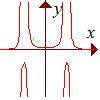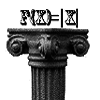Search by Topic

Resources tagged with Functions and their inverses similar to PCDF:

Filter by: Content type:
Age range:
Challenge level:

There are 13 results

Broad Topics > Functions and Graphs > Functions and their inversesPCDF

Age 16 to 18 Challenge Level:

When can a pdf and a cdf coincide?Inverting Rational Functions

Age 16 to 18 Challenge Level:

Consider these questions concerning inverting rational functionsAge 16 to 18 Challenge Level:

Decipher a simple code based on the rule C=7P+17 (mod 26) where C is the code for the letter P from the alphabet. Rearrange the formula and use the inverse to decipher automatically.Rational Request

Age 16 to 18 Challenge Level:

Can you make a curve to match my friend's requirements?Function Pyramids

Age 16 to 18 Challenge Level:

A function pyramid is a structure where each entry in the pyramid is determined by the two entries below it. Can you figure out how the pyramid is generated?Maths Shop Window

Age 16 to 18 Challenge Level:

Make a functional window display which will both satisfy the manager and make sense to the shoppersDouble Time

Age 16 to 18 Challenge Level:

Crack this code which depends on taking pairs of letters and using two simultaneous relations and modulus arithmetic to encode the message.Area L

Age 16 to 18 Challenge Level:

By sketching a graph of a continuous increasing function, can you prove a useful result about integrals?Pitchfork

Age 16 to 18 Challenge Level:

Plot the graph of x^y = y^x in the first quadrant and explain its properties.Real-life Equations

Age 16 to 18 Challenge Level:

Here are several equations from real life. Can you work out which measurements are possible from each equation?Curve Match

Age 16 to 18 Challenge Level:

Which curve is which, and how would you plan a route to pass between them?Equation Matcher

Age 16 to 18 Challenge Level:

Can you match these equations to these graphs?Modular Fractions

Age 16 to 18 Challenge Level:

We only need 7 numbers for modulus (or clock) arithmetic mod 7 including working with fractions. Explore how to divide numbers and write fractions in modulus arithemtic.